## 24 Feb What are the 16 Basic Shapes?We may not realize this, but shapes are all around us. We come across items of every shape in our home, out on the streets and in nature. Shapes can be a rectangular prism building, a sphere shape football, the crescent moon during a certain time of the month and even the oval-shaped of our eyes.

Children are first introduced to shapes as young children at a preschool level. In primary school, they learn about geometric shapes such as the circle, square, triangle and etcetera. At the most basic level, they are first taught how to identify and name the shapes, they will then be exposed to calculating the perimeter and area (2D shapes) and volume (3D shapes) using the specific formula for each shape.

After mastering the above calculations children will then be taught to apply them in problem-solving questions involving composite shapes (more than one shape) and real-world scenarios.

When it comes to questions involving real-world scenarios your child may find it difficult at first to apply the basic calculations that they have learned into more complex questions that require some imagination on their side.

We have compiled a list of the 16 basic shapes for your child to get to know and where they may be able to spot them.

## The Circle

The circle is very easily identified in the world around your child. It could be the clock on the wall, a classmate’s harry potter style glasses, a merry go round in the park, a roundabout on the road and a full moon which appears once a month.

A semicircle is a circle that has been separated into half.

Formula:

Circle

Circumference = 2πr Area = πr2

Semicircle

Perimeter=  ½ (2πr) + 2r

Area=  ½πr2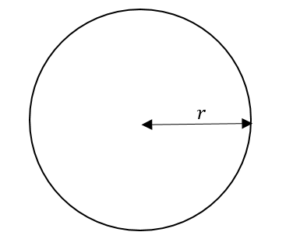## The Oval/Ellipse

Although not as commonly spotted as the circle, your child will be able to spot the oval shape in wall and handheld mirrors, in fruits such as the watermelon and the kiwi and even the oval-shaped of eggs. Another classic example of an oval-shaped object would be the ball used in American football.

## The Square

Children will certainly be familiar with the cartoon character ‘Spongebob Squarepants’ and his pair of iconic pants. The square shape is easily identifiable as one of the most common shapes for everyday items such as tables, photo frames and most biscuits that your child will snack on.

Formula

Length of sides = l

Perimeter = l + l + l + l = 4l

Area = l × l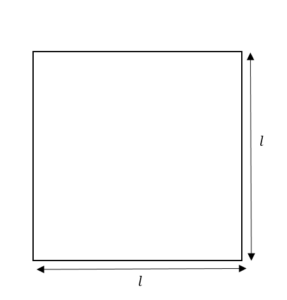## The Rectangle

Take your child on a grocery shopping trip and they are bound to see rectangular packaging of all sizes for food and other household items on the display shelves. Your child’s textbooks and exercise books are almost always rectangular in shape. Another rectangle shape item that your child may be familiar with would be the screens on the smartphone and computers.

Formula:

Length of sides = x (width) and y (length)

Perimeter=  2x + 2y

Area= x × y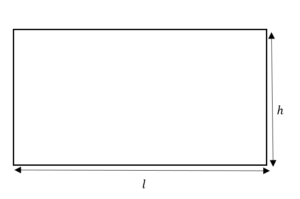## The Triangle

Your kids may be familiar with a triangular instrument often seen in movie scenes depicted an orchestra or music lesson, the triangle which is a kind of percussion is an interesting piece of instrument used by classical musicians. Other examples of triangles in our daily lives sails on a boat which helps to stir the boarding against and along the direction of the wind.

Formula:

Height of triangle = h

Base of triangle = B

Length of sides = l

Perimeter= sum of length of sides

Area=  ½ × h × B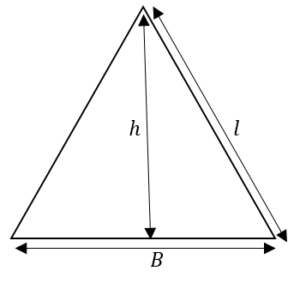## The Rhombus/Diamond

The Rhombus or same may also call it the diamond is a shape with four sides that has opposite angles that are equal, unlike the square which has four right angles. The rhombus shape can often be seen in kites and also the diamond shape in playing cards.

Formula:

Diameter of rhombus=d1 and d2

Perimeter= sum of length of sides

Area= =  ½ × d1 × d2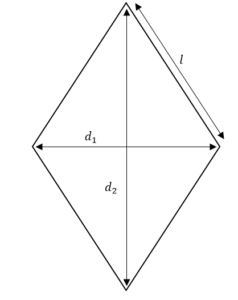## The Trapezium

The trapezium has four side. Its top and bottom bases are parallel while its sides are usually not parallel. Some examples of trapezium objects are the popcorn boxes used by the cinema and ladies’ handbags which are usually trapezoid in shape.

Formula:

Length of sides = l1 + l2

height of trapezium = h

Perimeter = sum of length of sides

Area =  ½ × ( l1  +l2 ) × h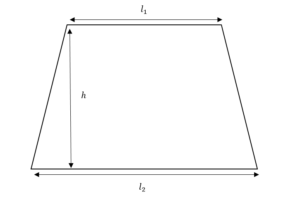## The Pentagon (Polygon with 5 sides)A pentagon is a polygon that has five sides. The pentagon can be symmetrical (the length of its sides and interior angles are the same) or asymmetrical (different side lengths and interior angles).

One very famous landmark in the USA is shaped and named after the pentagon, located in Arlington County in the state of Virginia, The Pentagon is the headquarters of the US Department of Defence. A birdhouse where birds cans seek shelter can also be built in the shape of a pentagon.

Formula:

Perimeter= sum of length of sides

Area= ½ x perimeter x apothem (length of midpoint of side to centre of circle)

Exterior angle of a regular pentagon =  360° / 5  = 72 o

Sum of Interior angle of a regular pentagon = (5-2) x 180 = 540 o interior angle =  540° / 5  = 108 o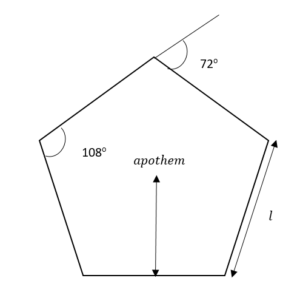## The Hexagon (Polygon with 6 sides)

Of similar character as the pentagon, the hexagon can be spotted in nature inside a honeycomb. Honey bees construct hexagonal honeycombs as this is the most effective way for them to store their honey. Pencils and colour pencils also have a hexagonal shape, this ensures that the pencils can be efficiently stacked up for storage and will not easily fall off the table.

Formula:

Perimeter =  sum of length of sides

Area= ½ x perimeter x apothem (length of midpoint of side to centre of circle)

Exterior angle of a regular pentagon =  360 o / 6  = 60 o

Sum of Interior angle of a regular hexagon = (6-2) x 180 = 720 o , interior angle =   720° / 6  = 120 o## The Octagon (Polygon with 8 sides)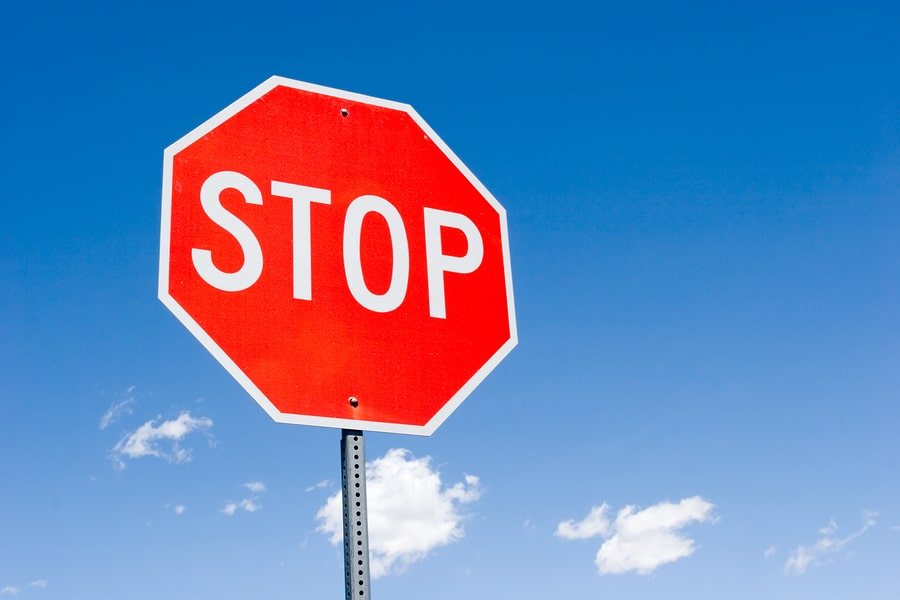The octagon is an eight-sided polygon that is less commonly used and seen compared to the hexagon and pentagon. The octagon is normally used in the design of windows and tiles for aesthetic effect. However, one octagonal-shaped object that we may see around is the stop sign on the road.

Formula:

Perimeter= sum of length of sides

Area= ½ x perimeter x apothem (length of midpoint of side to centre of circle)

Exterior angle of a regular pentagon =  360 o / 8  = 45 o

Sum of Interior angle of a regular octagon = (8-2) x 180 o = 1080  o , interior angle =  1080 ° / 8  = 135 o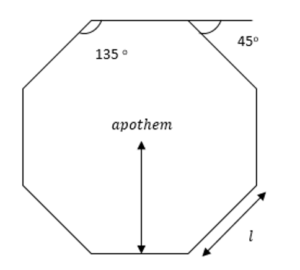## The Cylinder

A cylinder is a 3D solid or hollow object with a curved surface and circles at the side of both ends

Some examples of solid cylinders we see in our daily lives are batteries and canned food and soft drinks. Hollow cylinders are those that are hollow on their insides such as the pots and pans used for cooking and also buckets.

Formula:

Height of cylinder = h

Base of cylinder (circle) = B = πr2

Solid cylinder

Total surface area = 2πrh × 2 πr2  Volume= B × h

Hollow cylinder

Total surface area = lateral surface area (external + internal) + areas of solid bases

= (2πr1 h + 2πr2 h ) +  2π (r12-r22 )

Volume= Volume of external cylinder – Volume of internal cylinder

Volume = B1 × h – B2 × h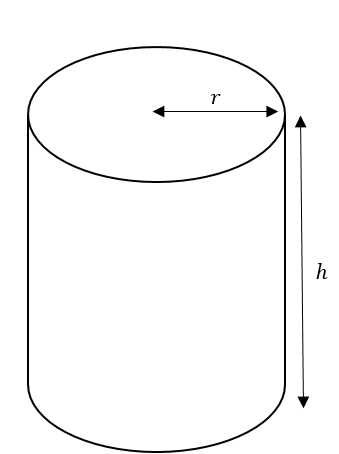## The Cone

A cone is a geometrical solid with a round base and only one vertex. Some commonly seen conical objects are the orange traffic cone and also the ever-popular ice cream cone.

Formula:

Height of cone = h

Slant height = s

Total surface area= lateral surface area + area of circle base

TSA = πrs + πr2

Volume =  1/3 πr2h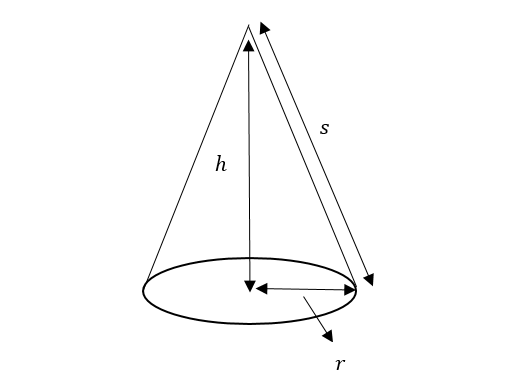## The Cube

The cube is essentially a solid made out of six square surfaces. Some examples of the cube are the Rubik’s cube consists of 27 smaller cubes inside one big cube. Dices used during board games are also cubes.

Formula:

Total surface area =  6 × area of square

Volume = width × length × height = w + l + h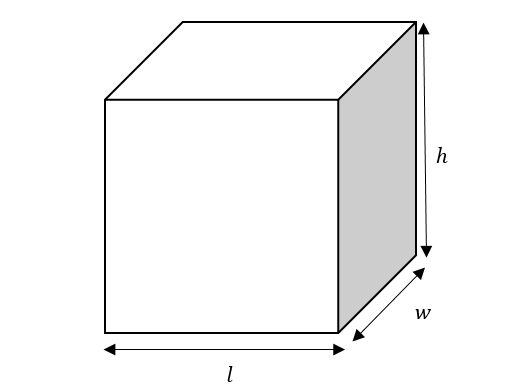## The Cuboid/Rectangular Prism

Similar to the cube a cuboid is a solid made out of six rectangular faces. Some cuboid objects that your child may come across are Legos in a Lego set, shoe boxes when purchasing new shows or even the microwave at home.

Formula:

Total surface area = Sum of area of each rectangle

Volume = width × length × height = w + l + h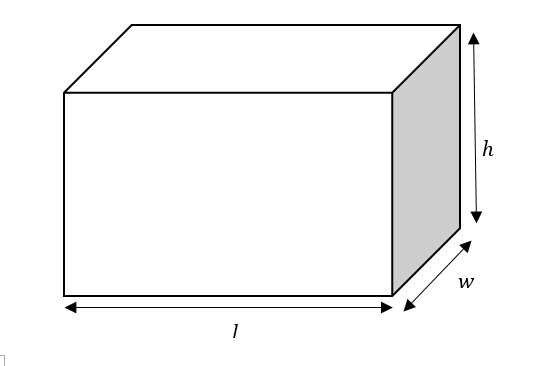## The Pyramid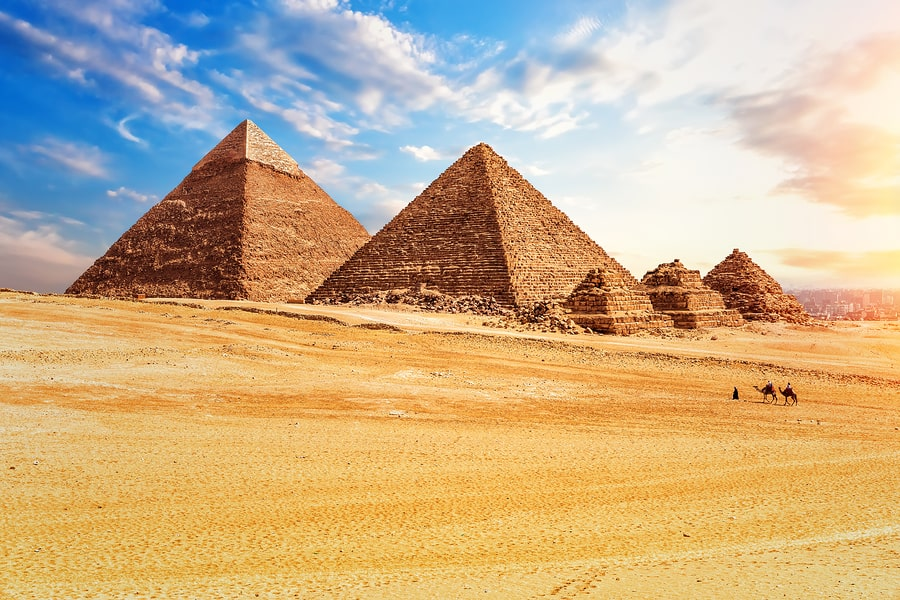The Great Pyramid of Giza was a great feat of architecture built by the ancient Egyptians. The Egyptian builders constructed the pyramid one block at a time with no room for error. The pyramid is a square-based pyramid. There are also pyramids that have triangular and rectangular bases.

Formula:

Total surface area = Area of 4 triangle surface + area of base (square/rectangle)

OR Sum of area of 4 triangle surface (for triangular based pyramid)

Volume =  1/3  × area of base (square/rectangle/triangle) × h, height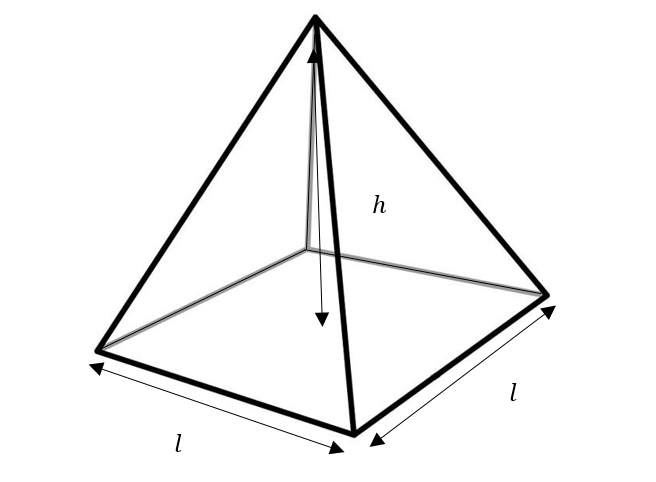## The Sphere

Our earth is a sphere as can be seen from outer space and also through a model of a globe. The calculation of points of location on the earth using longitude and latitude is based on the degree of a circle.  The other planets are also spherical in shape.

Other sphere shape items are balls of all sizes and functions such as the soccer ball, volleyball, basketball and golf ball.

A hemisphere is a sphere that has been cut into half.

Formula:

Sphere Total surface area = 4πr2

Volume =  4/3 πr3

Hemisphere Total surface area = 3πr2

Volume =  2/3 πr3